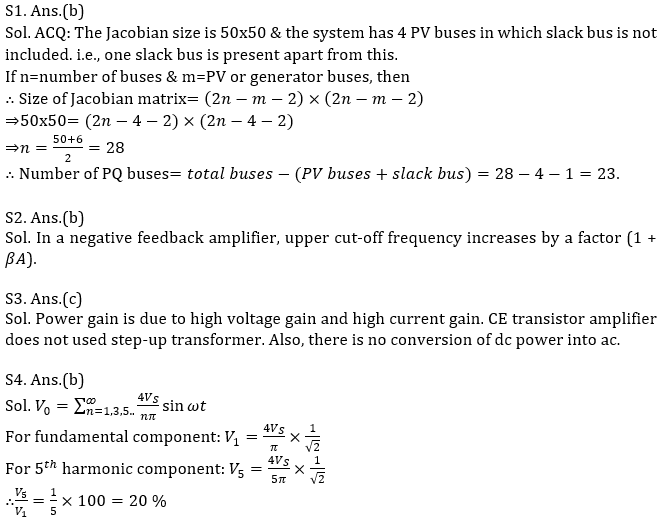Engineering Jobs   »   GATE ELECTRICAL QUIZ, GATE 2022 ELECTRICAL

# GATE’22 EE: Daily Practices Quiz 30-July-2021

Each question carries 2 marks
Negative marking: 0.66 mark

Total Questions: 05
Total marks: 10
Time: 12 min.

Q1. A power flow problem is solved using Newton-Raphson power flow in polar coordinate. The Jacobian size is 50×50. There is one slack bus in the system. The number of PV buses is 4. Find the number of PQ buses?
(a) 22
(b) 23
(c) 24
(d) 26

Q2. A negative feedback amplifier with open-loop gain (-A_0)/(1+j ω/ω_0 ) ;A_0>0 and feedback factor β(>0) will have a 3dB cutoff at what frequency?
(a) ω_0 A_0 β
(b) ω_0 (1+A_0 β)
(c) ω_0/((1+A_0 β) )
(d) ω_0 (1+A_0 β)

Q3. Consider the following with reference to a CE transistor amplifier
1. The use of negative feedback.
2. The conversion of dc power to ac.
3. High voltage and current gains.
4. The use of step-up transformer.
The power gain is due to
(a) 1 and 2
(b) 2 and 3
(c) 1 and 3
(d) 1 and 4.

Q4. A single-phase inverter has square wave output voltage. What is the percentage of the 5^th harmonic component in relation to the fundamental component?
(a) 10 %
(b) 20 %
(c) 30 %
(d) 40 %

Q5. A synchronous machine having E=1.1 pu is feeding an infinite bus with voltage of 1 pu, if the transfer reactance is 0.5 PU, the steady state power limit is
(a) 1.1 pu
(b) 0.55 pu
(c) 2.2 pu
(d) 2.6 pu

SOLUTIONSS5. Ans.(c)
Sol. P=EV/X_pu =(1.1 ×1)/0.5=2.2 pu

Sharing is caring!